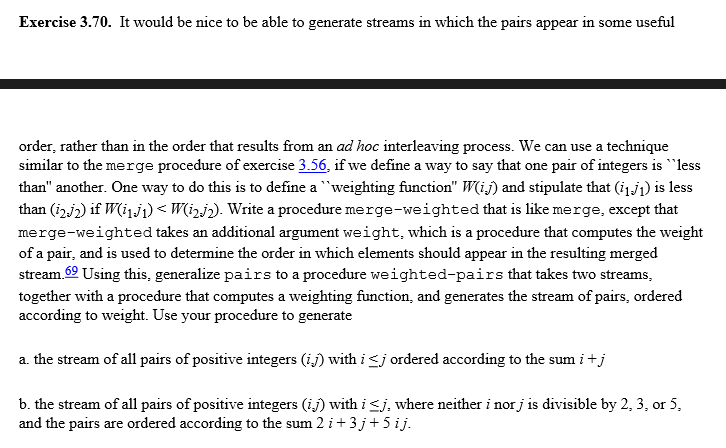2015/09/08 21:48

# 练习3-70

## 原文## 代码

(define (merge-weighted s1 s2 weight) (cond ((stream-null? s1) s2) ((stream-null? s2) s1) (else (let ((cars1 (stream-car s1)) (cars2 (stream-car s2))) (cond ((< (weight cars1) (weight cars2)) (cons-stream cars1 (merge-weighted (stream-cdr s1) s2 weight))) ((= (weight cars1) (weight cars2)) (cons-stream cars1 (merge-weighted (stream-cdr s1) s2 weight))) (else (cons-stream cars2 (merge-weighted s1 (stream-cdr s2) weight))))))))

(define (weighted-pairs s1 s2 weight) (cons-stream (list (stream-car s1) (stream-car s2)) (merge-weighted (stream-map (lambda (x) (list (stream-car s1) x)) (stream-cdr s2)) (weighted-pairs (stream-cdr s1) (stream-cdr s2) weight) weight)))

(define weight1 (lambda (x) (+ (car x) (cadr x))))

(define pairs1 (weighted-pairs integers integers weight1))

(define weight2 (lambda (x) (+ (* 2 (car x)) (* 3 (cadr x)) (* 5 (car x) (cadr x)))))

(define (divide? x y) (= (remainder y x) 0))

(define stream235 (stream-filter (lambda (x) (not (or (divide? 2 x) (divide? 3 x) (divide? 5 x)))) integers))

(define pairs2 (weighted-pairs stream235 stream235 stream235 weight2))



http://blog.csdn.net/nomasp

0
0 收藏

### 作者的其它热门文章0 评论
0 收藏
0